Function Repository Resource:

# HessianLine

Get the Hessian normal form of a line passing through two given points on the Cartesian plane

Contributed by: Ed Pegg Jr
 ResourceFunction["HessianLine"][pts] returns the Hessian line for pts.

## Details

Let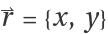and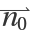be the normal vector {a,b}, such that a2+b2=1. The Hessian normal form of a line is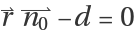, with d the distance from the origin.
The Hessian normal form a x+b y-d=0 can also be written as {a,b,d}.{x,y,-1}=0. ResourceFunction["HessianLine"] returns the triple {a,b,d}.

## Examples

### Basic Examples (2)

Find the Hessian line through two points:

 In:=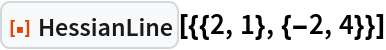Out=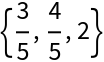Show the plane, points, origin and normal vector:

 In:=Out=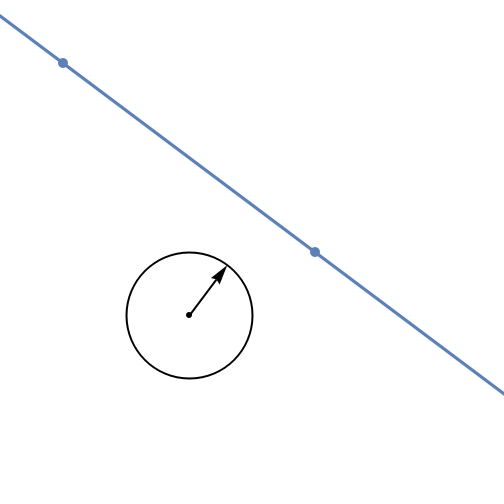### Scope (1)

Use HessianLine with symbolic arguments:

 In:=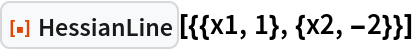Out=### Possible Issues (1)

HessianPlane fails if the given points are identical:

 In:=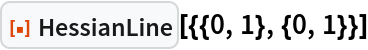Out=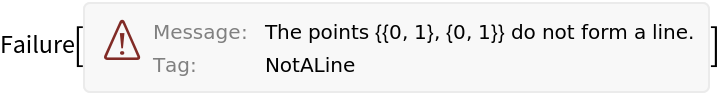### Neat Examples (3)

Find the Hessian lines through four points:

 In:=Out=The lines are distinct, so no three points are on a line:

 In:=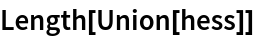Out=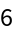Two of the lines have the same normal vector, so they are parallel:

 In:=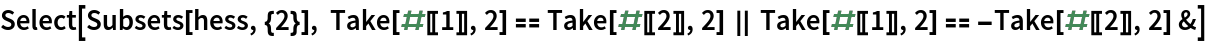Out=## Version History

• 1.0.0 – 09 December 2022

## Author Notes

Wanted to write a TrapezoidQ function, figured I should finish this first.